# VLookup Week at ExcelDashboardTemplates.com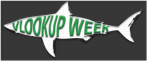The Awesome Mr. Excel has started a VLOOKUP week.  Much like Shark Week, all the Excel sites around the web are posting VLOOKUP learning posts to help users from stop fearing the use of lookup formulas.

What is VLOOKUP?  It is a way to search for a value in a list and return a corresponding value in another column in the list.

For instance, say you have a list of data as such: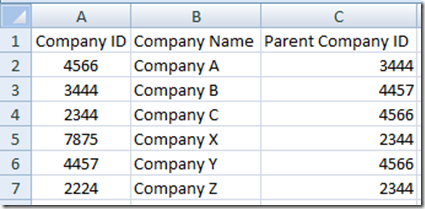And you want to find the parent company name for each account.  This is a perfect case for VLOOKUP.  It is a perfect VLOOKUP formula candidate because the lookup list left most column starts with the values that you want to find and then the value you want to return is to the right of that column.

Here are the details that you need to provide in the VLOOKUP formula: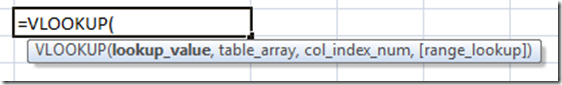For the VLOOKUP function, you will need to provide the Lookup Value that you want to search for in the left most column of a bigger list (called Table Array) and it will return another value from a different related column from the bigger list based on your Column Index value and finally you can specify if the formula needs to be an exact match or an approximate match in the Range Lookup section.

Here is what this VLOOKUP formula will look like (Note I have omitted the Range Lookup value and therefore the system will default it to an Exact Match):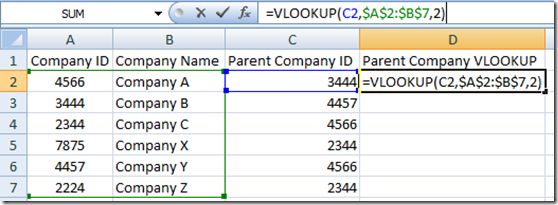and here is the resulting VLOOKUP for all rows of data: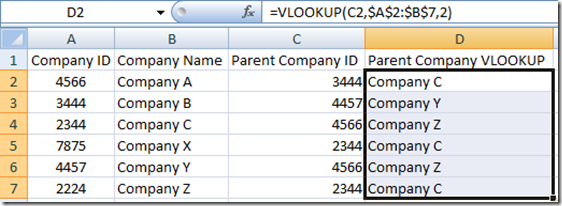You can see the power of the VLOOKUP Function to match data and return other related information from the lookup.  Although VLOOKUP function is great and simple to use, sometimes it can be very cumbersome to use because your lookup value will only lookup in the Left most column of your look up array.  This is the biggest drawback of the VLOOKUP function.  What if your data is set up in such a way that the Company Name is to the LEFT of the Company ID?  VLOOKUP WILL NOT WORK.  Here is how this data set may look: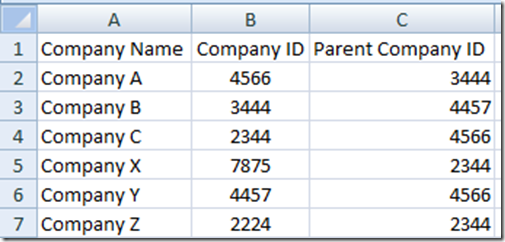In this case VLOOKUP is not the best solution to as it cannot lookup left.

Now you may ask yourself: “Steve=True, why don’t you just copy the Company ID to column A or left of the data that you want to return in your VLOOKUP?”

I would then reply that sometimes this is not feasible or wanted.  Perhaps you are regularly downloading the data from another system and the Lookup Value is to the right of your data you want to return.  Or perhaps you are restricted from doing this from your company.  Or perhaps you have a large data set that you want to lookup and return many different columns and having one large array will slow down your worksheet calculations.  How can we solve this?  We need a LEFT VLOOKUP Formula!!

How-To Create a LEFT VLOOKUP Function

Recently for a client, I had 393,000 records in my lookup array and because we were doing many different downloads and tests and it was not feasible to always shift the lookup list column to the left side of the lookup array.  Therefore, I needed to know how to create a LEFT VLOOKUP Function.

How can this be done?

I prefer to use a combination of Excel’s Index and Match functions to create the Left VLOOKUP.

Why would I use this in Excel Company Dashboard development?

# If you found the website and tutorials helpful, please consider donating to keep the lights on.

## Donate with PayPal here:You may have the need to transform your raw data into different categories or groupings that you will then use in your Executive Dashboards.  These lookups and translation to a new data grouping can easily be performed by using these lookup type functions.

Here is how the Spreadsheet would look using a combination Excel Index/Match Formula for a Left VLOOKUP: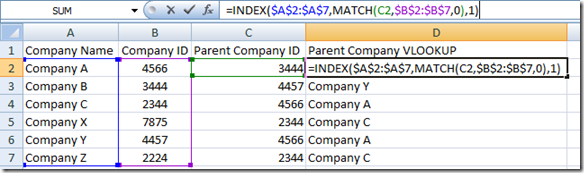So look at that, you can do a Left VLOOKUP in Excel, but it involves a bit of trickery because you are using a combination of Index Formula and Match Formula.

This topic deserves a more in-depth post on how to really use Index/Match as a VLOOKUP, so that will be my next post, because once you understand how to use a combined INDEX and Match Function, then you will almost never go back to using the VLOOKUP formula in Excel

See you in the next post on VLOOKUP WEEK for a detailed look at Index/Match for a Left VLookup.  It is really a great combination formula that you can use as a lookup formula, Up and Down Hlookup Formula as well as a Left and Right VLookup Formula.  Like in Lord of the Rings, this is one lookup formula to rule them all!

Please let me know what troubles you are having with any lookup type formulas and functions in Excel in the comments and please remember to sign up for the RSS feed so that you get the next installment of the LEFT VLOOKUP posting.

Steve=True